# How to use the F-Test in Microsoft Excel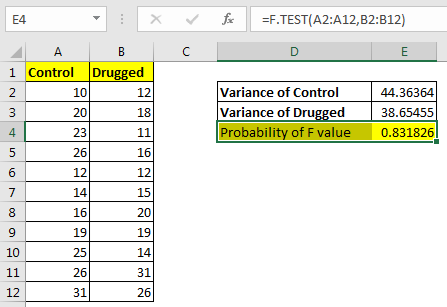In this article, we will learn How to use F-Test in Microsoft Excel.

What is the F-test function ?

The F.TEST Function is used to calculate F statistic of two samples in excel internally and returns the two tailed probability of the F statistic under Null Hypothesis (H0). Note that the F.TEST function does not return the F test value, instead it returns it’s probability. If F.TEST returns value less than 0.05, we reject the null hypothesis.

F.TEST function Syntax

 =F.TEST(array1, array2)

array1 : first sample of values. Mostly control samples.

array2 : second sample of values.

Example :

All of these might be confusing to understand. Let's understand how to use the function using an example. Here I have some data, where some students were given a drug and some don’t. I compared there data and evaluated the variance in each group.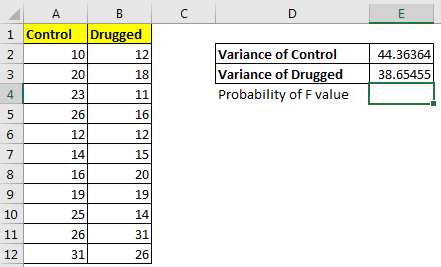We can see the difference in variance. But we want to check the probability of it. If it is less than 0.05 we will say there is a difference in variances else we will say there is no significant difference in variances of two groups.

Using the F.TEST function, we calculate the two tailed probability.

 =F.TEST(A2:A12,B2:B12)

It returns 0.83, which is much greater than 0.05. Hence we can’t reject the null hypothesis.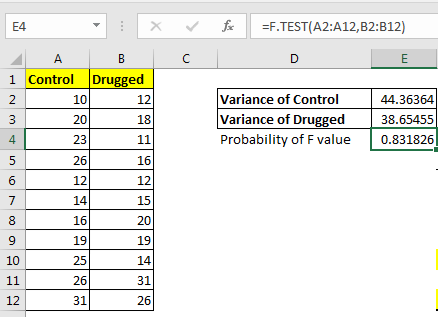How it works?

Let’s see how would we have got  F Test of data analysis.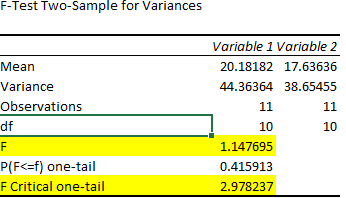And this how we would have interpreted it.

Well, if we calculate the F statistic (greater variance / smaller variance) we get 1.147. The critical value is 2.978. (Calculated using data analysis tool in excel). We can see that F value is less then F critical value, hence we can’t reject the null hypothesis.

This same thing we did just using one function F.TEST in excel. If the value is greater than 0.05 then we don’t reject the null hypothesis else we reject it.

Accessing F.TEST tool from Data Analysis Tool pack

To access this tool, click on Data Tab, click on Data Analysis in the Analysis group. If the Data Analysis command is not available then you need to load the Analysis Toolpak.

• Click the File Tab, Click options and click on Add INS Category.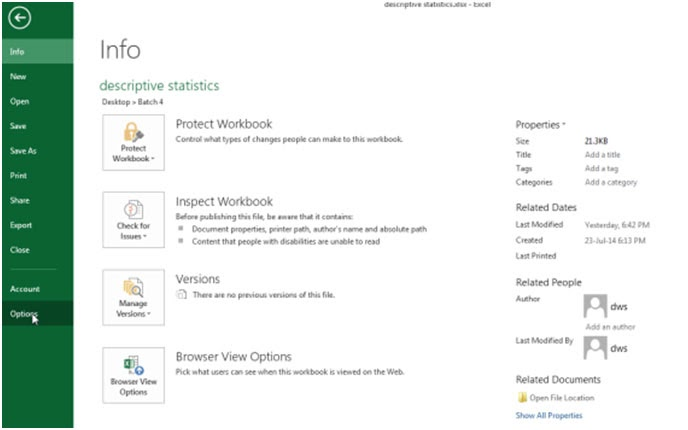In the Manage box, select the Analysis Toolpak and then click on the Go button.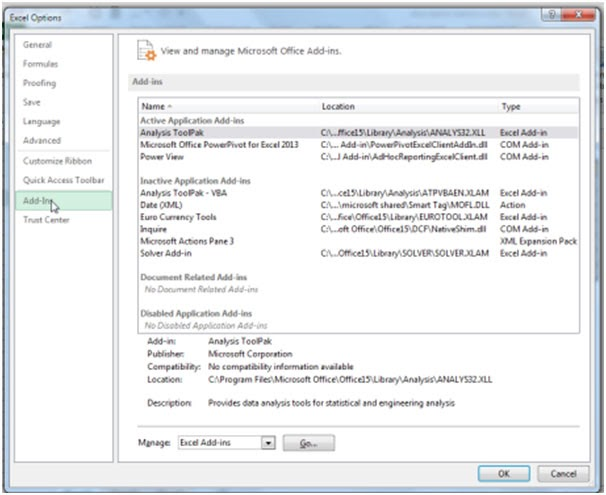• In the Add Ins dialog box, select the Analysis Toolpak check box and then click Ok.
• If Analysis Toolpak is not listed in the Add Ins available box, click Browse to locate it.
•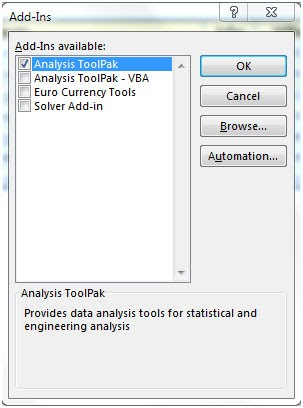Let’s come back to the topic, to analyze the data through F-Test Two-Sample for Variances.

We have remarks score data of 2 groups in the range A2:B12. Column A contains the data of Group 1 and column B contains the data of 2nd Group.

To use the F-Test Two-Sample for Variances, follow below mentioned steps:-

• Go to Data Tab.
• Click on Data Analysis in the Analyses group.
•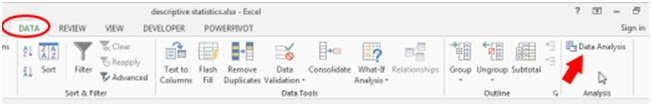• Data Analysis dialog box will appear.
•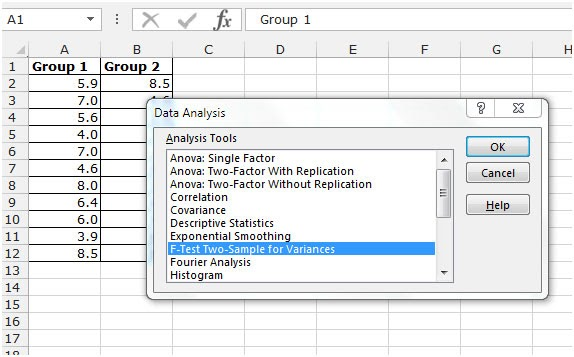• From the Analysis tool drop down menu, F-Test Two-Sample for Variances and click on ok.
• You will get F-Test Two-Sample for Variances dialog box will appear.
• Click on Input range. Select the 1st variable range A1:A12, select the 2nd variable range B1:B12.
• Tick on Labels in the first row to get the header in the result.
• Tick on Output Range and select the cell where you want to show the summary.
• Click on ok.
•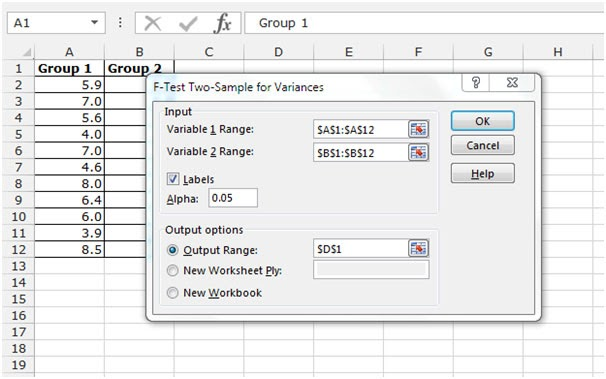•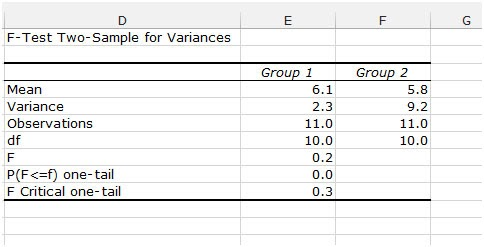Important: be sure that the variance of Variable 1 is higher than the variance of Variable 2. This is the case, 2.3<9.2. If not, swap your data. As a result, Excel calculates the correct F value, which is the ratio of Variance 1 to Variance 2 (F = 2.3/9.2= 0.25)

Hope this article about How to use the F-Test in Microsoft Excel is explanatory. Find more articles on calculating values and related Excel formulas here. If you liked our blogs, share it with your friends on Facebook. And also you can follow us on Twitter and Facebook. We would love to hear from you, do let us know how we can improve, complement or innovate our work and make it better for you. Write to us at info@exceltip.com.

Related Articles :

How To Use Excel T TEST Function in Excel : The T.TEST is used to determine the confidence of an analysis. Mathematically, it is used to know if the mean of the two samples are equal or not. T.TEST is used to accept or reject the null hypothesis.

How to use the DEVSQ Function in Excel : DEVSQ function is a built-in statistical function to calculate the sum of squared deviations from the mean or average of the range of data values provided.

How to use Excel NORM.DIST Function : Calculate the Z score for the normal cumulative distribution for the pre specified values using the NORMDIST function in Excel.

How to use Excel NORM.INV Function : Calculate the inverse of Z score for the normal cumulative distribution for the pre-specified probability values using the NORM.INV function in Excel.

How to Calculate Standard Deviation in Excel : To calculate the standard deviation we have different functions in Excel. The standard deviation is the square root of the variance value but It tells more about the dataset than variance.

How to use the VAR function in Excel : Calculate the variance for the sample dataset in excel using the VAR function in Excel.

Popular Articles :

How to use the IF Function in Excel : The IF statement in Excel checks the condition and returns a specific value if the condition is TRUE or returns another specific value if FALSE.

How to use the VLOOKUP Function in Excel : This is one of the most used and popular functions of excel that is used to lookup value from different ranges and sheets.

How to use the SUMIF Function in Excel : This is another dashboard essential function. This helps you sum up values on specific conditions.

How to use the COUNTIF Function in Excel : Count values with conditions using this amazing function. You don't need to filter your data to count specific values. Countif function is essential to prepare your dashboard.

Terms and Conditions of use

The applications/code on this site are distributed as is and without warranties or liability. In no event shall the owner of the copyrights, or the authors of the applications/code be liable for any loss of profit, any problems or any damage resulting from the use or evaluation of the applications/code.